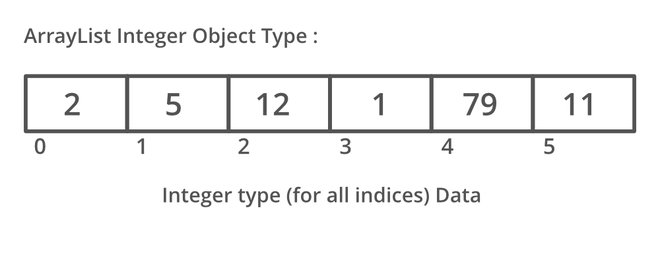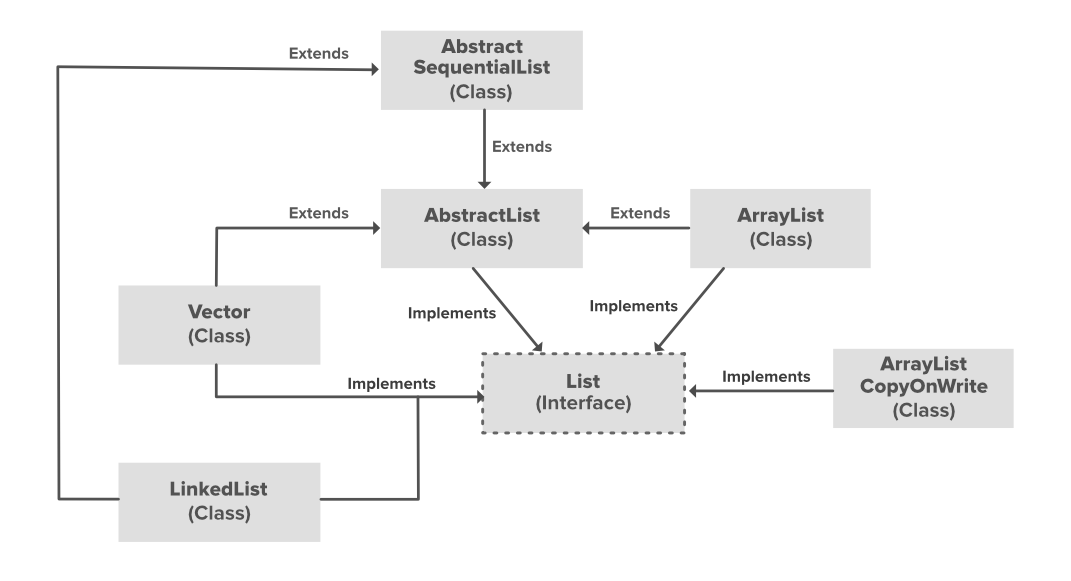# ArrayList in Java

• Difficulty Level : Easy
• Last Updated : 13 May, 2022

ArrayList is a part of collection framework and is present in java.util package. It provides us with dynamic arrays in Java. Though, it may be slower than standard arrays but can be helpful in programs where lots of manipulation in the array is needed. This class is found in java.util package.Illustration:Example: The following implementation demonstrates how to create and use an ArrayList.## Java

 `// Java program to demonstrate the  ` `// working of ArrayList in Java  ` ` `  `import` `java.io.*;  ` `import` `java.util.*;  ` ` `  `class` `ArrayListExample {  ` `    ``public` `static` `void` `main(String[] args)  ` `    ``{  ` `        ``// Size of the  ` `        ``// ArrayList  ` `        ``int` `n = ``5``;  ` ` `  `        ``// Declaring the ArrayList with  ` `        ``// initial size n  ` `        ``ArrayList arrli  ` `            ``= ``new` `ArrayList(n);  ` ` `  `        ``// Appending new elements at  ` `        ``// the end of the list  ` `        ``for` `(``int` `i = ``1``; i <= n; i++)  ` `            ``arrli.add(i);  ` ` `  `        ``// Printing elements  ` `        ``System.out.println(arrli);  ` ` `  `        ``// Remove element at index 3  ` `        ``arrli.remove(``3``);  ` ` `  `        ``// Displaying the ArrayList  ` `        ``// after deletion  ` `        ``System.out.println(arrli);  ` ` `  `        ``// Printing elements one by one  ` `        ``for` `(``int` `i = ``0``; i < arrli.size(); i++)  ` `            ``System.out.print(arrli.get(i) + ``" "``);  ` `    ``}  ` `}  `

Output

```[1, 2, 3, 4, 5]
[1, 2, 3, 5]
1 2 3 5 ```

Since ArrayList is a dynamic array and we do not have to specify the size while creating it, the size of the array automatically increases when we dynamically add and remove items. Though the actual library implementation may be more complex, the following is a very basic idea explaining the working of the array when the array becomes full and if we try to add an item:

• Creates a bigger-sized memory on heap memory (for example memory of double size).
• Copies the current memory elements to the new memory.
• New item is added now as there is bigger memory available now.
• Delete the old memory.

Important Features:

• ArrayList inherits AbstractList class and implements the List interface.
• ArrayList is initialized by the size. However, the size is increased automatically if the collection grows or shrinks if the objects are removed from the collection.
• Java ArrayList allows us to randomly access the list.
• ArrayList can not be used for primitive types, like int, char, etc. We need a wrapper class for such cases.
• ArrayList in Java can be seen as a vector in C++.
• ArrayList is not Synchronized. Its equivalent synchronized class in Java is Vector.

Let’s understand the Java ArrayList in depth. Look at the below image:In the above illustration, AbstractList, CopyOnWriteArrayList, and the AbstractSequentialList are the classes that implement the list interface. A separate functionality is implemented in each of the mentioned classes. They are:

1. AbstractList: This class is used to implement an unmodifiable list, for which one needs to only extend this AbstractList Class and implement only the get() and the size() methods.
2. CopyOnWriteArrayList: This class implements the list interface. It is an enhanced version of ArrayList in which all the modifications(add, set, remove, etc.) are implemented by making a fresh copy of the list.
3. AbstractSequentialList: This class implements the Collection interface and the AbstractCollection class. This class is used to implement an unmodifiable list, for which one needs to only extend this AbstractList Class and implement only the get() and the size() methods.

### Constructors in the ArrayList

In order to create an ArrayList, we need to create an object of the ArrayList class. The ArrayList class consists of various constructors which allow the possible creation of the array list. The following are the constructors available in this class:

1. ArrayList(): This constructor is used to build an empty array list. If we wish to create an empty ArrayList with the name arr, then, it can be created as:

ArrayList arr = new ArrayList();

2. ArrayList(Collection c): This constructor is used to build an array list initialized with the elements from the collection c. Suppose, we wish to create an ArrayList arr which contains the elements present in the collection c, then, it can be created as:

ArrayList arr = new ArrayList(c);

3. ArrayList(int capacity): This constructor is used to build an array list with initial capacity being specified. Suppose we wish to create an ArrayList with the initial size being N, then, it can be created as:

ArrayList arr = new ArrayList(N);

### Methods in Java ArrayList

Note: You can also create a generic ArrayList:

```// Creating generic integer ArrayList
ArrayList<Integer> arrli = new ArrayList<Integer>();```

Let’s see how to perform some basics operations on the ArrayList as listed which we are going to discuss further alongside implementing every operation.

• Changing elements
• Removing elements
• Iterating elements

In order to add an element to an ArrayList, we can use the add() method. This method is overloaded to perform multiple operations based on different parameters. They are as follows:

• add(Object): This method is used to add an element at the end of the ArrayList.
• add(int index, Object): This method is used to add an element at a specific index in the ArrayList.

Example:

## Java

 `// Java Program to Add elements to An ArrayList ` ` `  `// Importing all utility classes ` `import` `java.util.*; ` ` `  `// Main class ` `class` `GFG { ` ` `  `    ``// Main driver method ` `    ``public` `static` `void` `main(String args[]) ` `    ``{ ` `        ``// Creating an Array of string type ` `        ``ArrayList al = ``new` `ArrayList<>(); ` ` `  `        ``// Adding elements to ArrayList ` `        ``// Custom inputs ` `        ``al.add(``"Geeks"``); ` `        ``al.add(``"Geeks"``); ` ` `  `        ``// Here we are mentioning the index ` `        ``// at which it is to be added ` `        ``al.add(``1``, ``"For"``); ` ` `  `        ``// Printing all the elements in an ArrayList ` `        ``System.out.println(al); ` `    ``} ` `}`

Output:

`[Geeks, For, Geeks]`

Operation 2: Changing Elements

After adding the elements, if we wish to change the element, it can be done using the set() method. Since an ArrayList is indexed, the element which we wish to change is referenced by the index of the element. Therefore, this method takes an index and the updated element which needs to be inserted at that index.

Example

## Java

 `// Java Program to Change elements in ArrayList ` ` `  `// Importing all utility classes ` `import` `java.util.*; ` ` `  `// main class ` `class` `GFG { ` ` `  `    ``// Main driver method ` `    ``public` `static` `void` `main(String args[]) ` `    ``{ ` `        ``// Creating an Arraylist object of string type ` `        ``ArrayList al = ``new` `ArrayList<>(); ` ` `  `        ``// Adding elements to Arraylist ` `        ``// Custom input elements ` `        ``al.add(``"Geeks"``); ` `        ``al.add(``"Geeks"``); ` ` `  `        ``// Adding specifying the index to be added ` `        ``al.add(``1``, ``"Geeks"``); ` ` `  `        ``// Printing the Arraylist elements ` `        ``System.out.println(``"Initial ArrayList "` `+ al); ` ` `  `        ``// Setting element at 1st index ` `        ``al.set(``1``, ``"For"``); ` ` `  `        ``//  Printing the updated Arraylist ` `        ``System.out.println(``"Updated ArrayList "` `+ al); ` `    ``} ` `}`

Output:

```Initial ArrayList [Geeks, Geeks, Geeks]
Updated ArrayList [Geeks, For, Geeks]```

Operation 3: Removing Elements

In order to remove an element from an ArrayList, we can use the remove() method. This method is overloaded to perform multiple operations based on different parameters. They are as follows:

• remove(Object): This method is used to simply remove an object from the ArrayList. If there are multiple such objects, then the first occurrence of the object is removed.
• remove(int index): Since an ArrayList is indexed, this method takes an integer value which simply removes the element present at that specific index in the ArrayList. After removing the element, all the elements are moved to the left to fill the space and the indices of the objects are updated.

Example

## Java

 `// Java program to Remove Elements in ArrayList ` ` `  `// Importing all utility classes ` `import` `java.util.*; ` ` `  `// Main class ` `class` `GFG { ` ` `  `    ``// Main driver method ` `    ``public` `static` `void` `main(String args[]) ` `    ``{ ` `        ``// Creating an object of arraylist class ` `        ``ArrayList al = ``new` `ArrayList<>(); ` ` `  `        ``// Adding elements to ArrayList ` `        ``// Custom addition ` `        ``al.add(``"Geeks"``); ` `        ``al.add(``"Geeks"``); ` `        ``// Adding element at specific index ` `        ``al.add(``1``, ``"For"``); ` ` `  `        ``// Printing all elements of ArrayList ` `        ``System.out.println(``"Initial ArrayList "` `+ al); ` ` `  `        ``// Removing element from above ArrayList ` `        ``al.remove(``1``); ` ` `  `        ``// Printing the updated Arraylist elements ` `        ``System.out.println(``"After the Index Removal "` `+ al); ` ` `  `        ``// Removing this word element in ArrayList ` `        ``al.remove(``"Geeks"``); ` ` `  `        ``// Now printing updated ArrayList ` `        ``System.out.println(``"After the Object Removal "` `                           ``+ al); ` `    ``} ` `}`

Output:

```Initial ArrayList [Geeks, For, Geeks]
After the Index Removal [Geeks, Geeks]
After the Object Removal [Geeks]```

Operation 4: Iterating the ArrayList

There are multiple ways to iterate through the ArrayList. The most famous ways are by using the basic for loop in combination with a get() method to get the element at a specific index and the advanced for loop

Example

## Java

 `// Java program to Iterate the elements ` `// in an ArrayList ` ` `  `// Importing all utility classes ` `import` `java.util.*; ` ` `  `// Main class ` `class` `GFG { ` ` `  `    ``// Main driver method ` `    ``public` `static` `void` `main(String args[]) ` `    ``{ ` `        ``// Creating an Arraylist of string type ` `        ``ArrayList al = ``new` `ArrayList<>(); ` ` `  `        ``// Adding elements to ArrayList ` `        ``//  using standard add() method ` `        ``al.add(``"Geeks"``); ` `        ``al.add(``"Geeks"``); ` `        ``al.add(``1``, ``"For"``); ` ` `  `        ``// Using the Get method and the ` `        ``// for loop ` `        ``for` `(``int` `i = ``0``; i < al.size(); i++) { ` ` `  `            ``System.out.print(al.get(i) + ``" "``); ` `        ``} ` ` `  `        ``System.out.println(); ` ` `  `        ``// Using the for each loop ` `        ``for` `(String str : al) ` `            ``System.out.print(str + ``" "``); ` `    ``} ` `}`

Output:

```Geeks For Geeks
Geeks For Geeks```

Must Read: Array vs ArrayList in Java

My Personal Notes arrow_drop_up
Recommended Articles
Page :